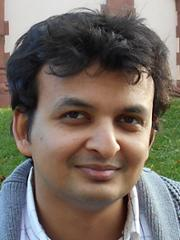Timezone: »

Poster
Learning to Collaborate in Markov Decision Processes

Tue Jun 11 06:30 PM -- 09:00 PM (PDT) @ Pacific Ballroom #105
We consider a two-agent MDP framework where agents repeatedly solve a task in a collaborative setting. We study the problem of designing a learning algorithm for the first agent (A1) that facilitates a successful collaboration even in cases when the second agent (A2) is adapting its policy in an unknown way. The key challenge in our setting is that the first agent faces non-stationarity in rewards and transitions because of the adaptive behavior of the second agent. We design novel online learning algorithms for agent A1 whose regret decays as $O(T^{1-\frac{3}{7} \cdot \alpha})$ with $T$ learning episodes provided that the magnitude of agent A2's policy changes between any two consecutive episodes are upper bounded by $O(T^{-\alpha})$. Here, the parameter $\alpha$ is assumed to be strictly greater than $0$, and we show that this assumption is necessary provided that the {\em learning parity with noise} problem is computationally hard. We show that sub-linear regret of agent A1 further implies near-optimality of the agents' joint return for MDPs that manifest the properties of a {\em smooth} game.

#### Author Information